# Colleges Still Accepting Applications for Fall 2019, Preparing for Summer at The College Map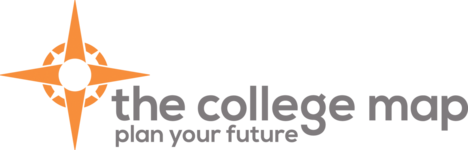# Hello, The College Map!

 table div table+table+table+table div table{width:100%;padding:0}table div table+table+table+table div table img{width:96.23%;padding:0;float:none}table div table+table+table+table div table td{width:100%;padding:0 1.88% 18px}/* styles */## Colleges Still Accepting Applications for Fall 2019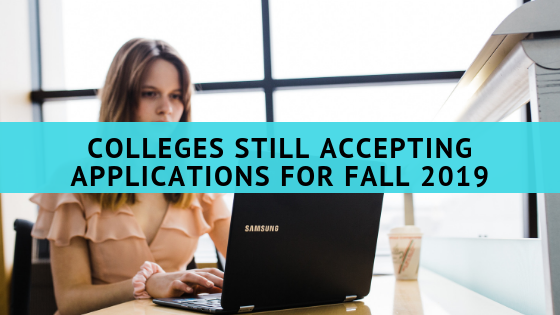/* styles */ Are you still looking for a college to attend in the fall? Maybe you did not get your tuition deposit in by May 1 and now the college doesn’t have a spot for you. Or, maybe your plans changed after realizing the college you wanted to attend was not a financial fit or too far away from home. Whatever the reason, there are many wonderful college still accepting applications for the fall. The National Association for College Admission Counseling (NACAC) recently released their annual College Openings Update: Options for Qualified Students. There are over 400 colleges currently on the list. Most of the colleges are still accepting freshman and transfer applicants and most will have financial aid and housing available for new students. The list of colleges still accepting applications is diverse. There are public and private colleges. There are small, medium, and large colleges. The list also has large research institutions, liberal arts colleges, and religious colleges. Some of the big names on the list include Baylor (Texas), Ithaca(New York), Arizona State University, and University of Oregon. Students still looking for a place to attend in the fall will surely find a college on the list that meets their requirements. As colleges and universities fill their spaces, they will close their applications. Therefore, if you’re still looking for a place to attend in the fall, you should apply to the institution as soon as possible. In addition, other colleges may join the list as they learn more about their incoming class and find they still have space available. If you’re still not sure where you will be attending in the fall, you still have options!
 table div table+table+table+table+table+table+table+table div table{width:100%;padding:0}table div table+table+table+table+table+table+table+table div table img{width:96.23%;padding:0;float:none}table div table+table+table+table+table+table+table+table div table td{width:100%;padding:0 1.88% 18px}/* styles */## Purposeful SummersWhile you may want to spend your entire summer sleeping in and hanging out with friends, it’s important also to spend part of the time being productive and preparing for your future. Participating in an activity over the summer can help you get a great job or get accepted to a top college in the future, and being productive over the summer doesn’t have to take up all your time or be boring!

Colleges and employers love seeing applicants who are motivated and interested in learning more. By keeping busy over the summer, you'll show them that you have what it takes to be a great addition to their school or workplace.

Here are a couple of ideas: VISIT COLLEGES, volunteer, take on a summer job, establish and/or clean up your online presence, internships, online classes, summer camps and staying active in your hobbies and interests.

 table div table+table+table+table+table+table+table+table+table+table+table div table{width:100%;padding:0}table div table+table+table+table+table+table+table+table+table+table+table div table img{width:96.23%;padding:0;float:none}table div table+table+table+table+table+table+table+table+table+table+table div table td{width:100%;padding:0 1.88% 18px}/* styles */## TCM Summer RemindersThe College Map will be closed every Saturday in June and July and Monday, July 1st - Sunday, July 7th

## Summer Hours and Important Dates

Saturdays in June and July:
CLOSED
(Test Prep only)

Monday, July 1st - Friday, July 7th:
CLOSED.
Happy Independence Day!

Thursday, July 18th:
UNF Apply in July Event.

Tuesday - Thursday, July 23rd - 25th & July 30th - August 1st:
Rising Senior Boot Camp

 table div table+table+table+table+table+table+table+table+table+table+table+table+table+table div table{width:100%;padding:0}table div table+table+table+table+table+table+table+table+table+table+table+table+table+table div table img{width:96.23%;padding:0;float:none}table div table+table+table+table+table+table+table+table+table+table+table+table+table+table div table td{width:100%;padding:0 1.88% 18px}/* styles */## Attention SAT Test Prep Students

 /* styles */ The May 11th SAT Verbal class time has changed. The class will now be taught from 8:30 a.m. to 10:30 a.m. This time change has been noted in Acuity.
 table div table+table+table+table+table+table+table+table+table+table+table+table+table+table+table+table+table div table{width:100%;padding:0}table div table+table+table+table+table+table+table+table+table+table+table+table+table+table+table+table+table div table img{width:96.23%;padding:0;float:none}table div table+table+table+table+table+table+table+table+table+table+table+table+table+table+table+table+table div table td{width:100%;padding:0 1.88% 18px}/* styles */## Rising Seniors Essay Workshop Reminder!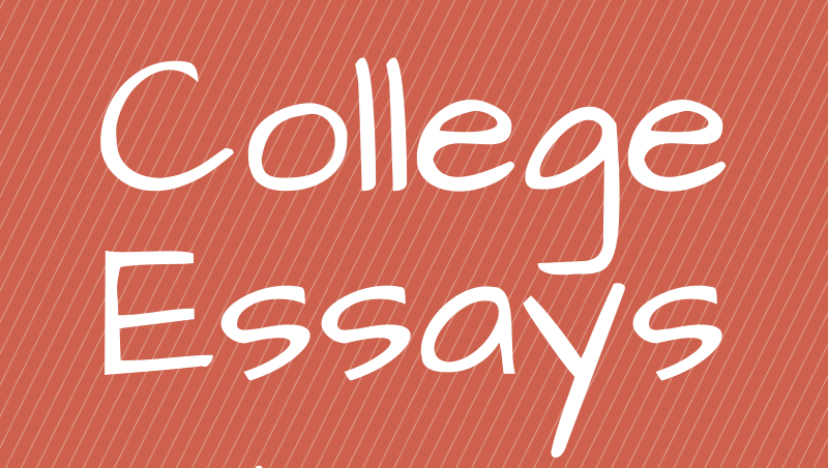/* styles */ ALL rising seniors should be attending either May 18th's or June 1st's workshop date. The start time is 10am. Several communications have been sent regarding this workshop and was discussed in depth at our parent meeting back in April. This is our student's first step in the essay writing process. Mrs. Mitchell will present on the philosophy, goals, and execution of a masterful essay highlight what a student's key message statement might look and sound like. Additionally, students will be given the handout that includes the prompts for the Common App and the Coalition. It will include the quick reference sheet on the "Dos and Don'ts" of the writing process. Each student will be asked to do a "brain dump" of possible essay topics that they could write on. Mrs. Mitchell will sign off on the topic for them to begin to write a first draft of their essay, or at least a first paragraph. The workshop will last two hours and students are expected to attend for the full length. Mrs. Mitchell and Mrs. Etscorn will be moving the essays forward and finalizing them through the boot camp dates in July. PLEASE make sure to attend!
 table div table+table+table+table+table+table+table+table+table+table+table+table+table+table+table+table+table+table+table+table+table div table{width:100%;padding:0}table div table+table+table+table+table+table+table+table+table+table+table+table+table+table+table+table+table+table+table+table+table div table img{width:96.23%;padding:0;float:none}table div table+table+table+table+table+table+table+table+table+table+table+table+table+table+table+table+table+table+table+table+table div table td{width:100%;padding:0 1.88% 18px}/* styles */## Apply In July to UNF!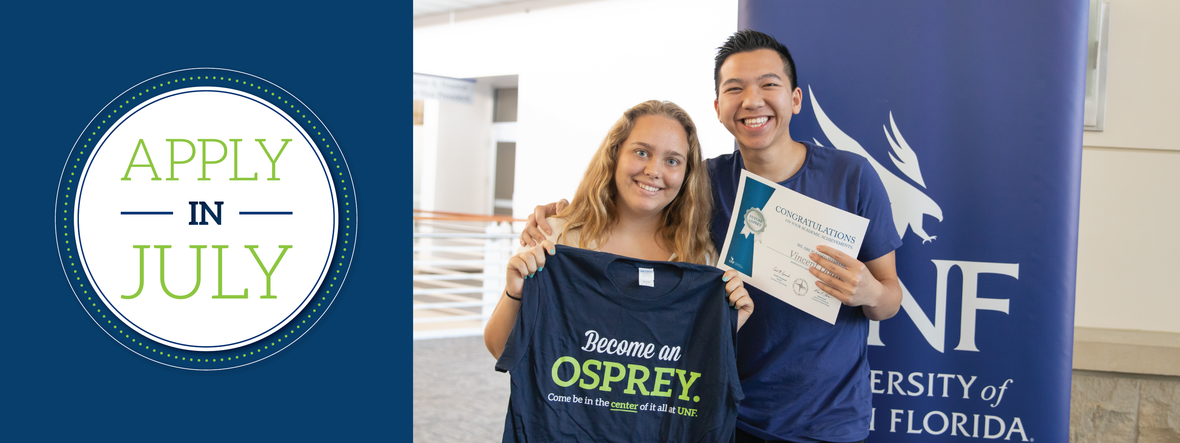table div table+table+table+table+table+table+table+table+table+table+table+table+table+table+table+table+table+table+table+table+table+table+table+table+table div table{width:100%;padding:0}table div table+table+table+table+table+table+table+table+table+table+table+table+table+table+table+table+table+table+table+table+table+table+table+table+table div table img{width:96.23%;padding:0;float:none}table div table+table+table+table+table+table+table+table+table+table+table+table+table+table+table+table+table+table+table+table+table+table+table+table+table div table td{width:100%;padding:0 1.88% 18px}/* styles */## Service Hours & Bright Futures!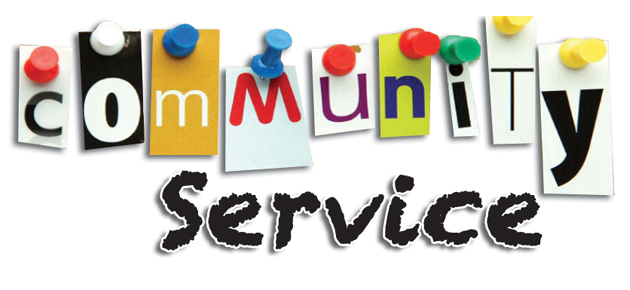table div table+table+table+table+table+table+table+table+table+table+table+table+table+table+table+table+table+table+table+table+table+table+table+table+table+table+table+table+table div table{width:100%;padding:0}table div table+table+table+table+table+table+table+table+table+table+table+table+table+table+table+table+table+table+table+table+table+table+table+table+table+table+table+table+table div table img{width:96.23%;padding:0;float:none}table div table+table+table+table+table+table+table+table+table+table+table+table+table+table+table+table+table+table+table+table+table+table+table+table+table+table+table+table+table div table td{width:100%;padding:0 1.88% 18px}/* styles */## Bright Futures Application!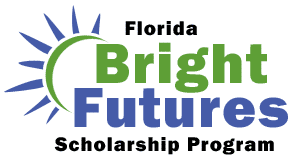/* styles */ Seniors, don't forget to submit a completed Florida Financial Aid Application (FFAA) in order to be considered for State Scholarship & Grant Programs. Seniors, please Create a Student Account. After logging into your account, you may proceed to complete the FFAA. The Bright Futures link can answer all of your questions: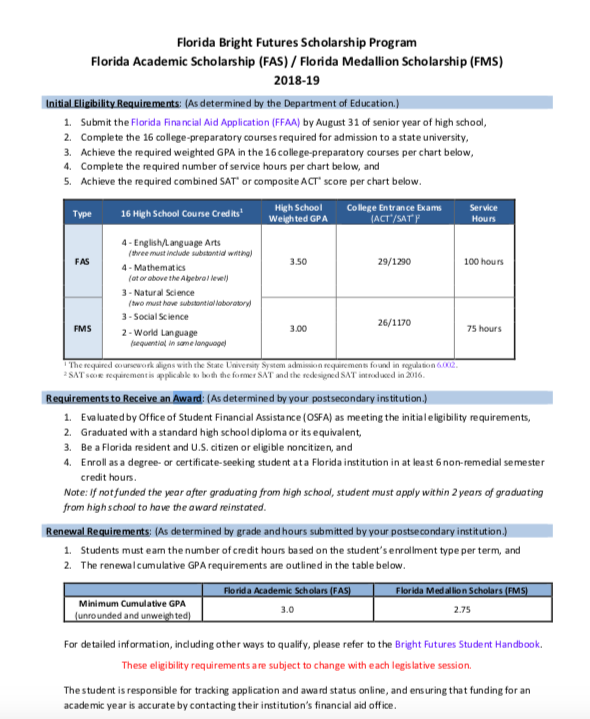table div table+table+table+table+table+table+table+table+table+table+table+table+table+table+table+table+table+table+table+table+table+table+table+table+table+table+table+table+table+table+table+table+table+table div table{width:100%;padding:0}table div table+table+table+table+table+table+table+table+table+table+table+table+table+table+table+table+table+table+table+table+table+table+table+table+table+table+table+table+table+table+table+table+table+table div table img{width:96.23%;padding:0;float:none}table div table+table+table+table+table+table+table+table+table+table+table+table+table+table+table+table+table+table+table+table+table+table+table+table+table+table+table+table+table+table+table+table+table+table div table td{width:100%;padding:0 1.88% 18px}/* styles */## SAT Subject Tests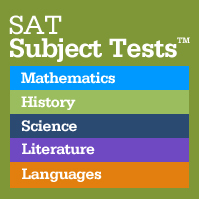/* styles */ SAT Subject Tests are subject specific college admissions exams. They are designed by the same company (College Board) that publishes the SAT and AP exams. There are twenty different subject tests available in the areas of English, Math, Science, and foreign language. They are one hour long multiple choice tests that are given on the dates that the SAT is given- but, not all twenty tests are offered on the same date. SAT Subject Tests are an admission data requirement for many elite, top schools. Most of these top schools require two subject test scores. Students in grades 10th and 11th should consider taking one, two, or three subject tests this June if they are "applying high." Strategically, it is best that a student plans to take a subject test in an area that they just completed an AP class and AP exam in. Students, we encourager you to reach out to your college planner if you have any questions about these tests. You must register for these tests just like you would register for the SAT at: www.collegeboard.org
 table div table+table+table+table+table+table+table+table+table+table+table+table+table+table+table+table+table+table+table+table+table+table+table+table+table+table+table+table+table+table+table+table+table+table+table+table+table+table div table{width:100%;padding:0}table div table+table+table+table+table+table+table+table+table+table+table+table+table+table+table+table+table+table+table+table+table+table+table+table+table+table+table+table+table+table+table+table+table+table+table+table+table+table div table img{width:96.23%;padding:0;float:none}table div table+table+table+table+table+table+table+table+table+table+table+table+table+table+table+table+table+table+table+table+table+table+table+table+table+table+table+table+table+table+table+table+table+table+table+table+table+table div table td{width:100%;padding:0 1.88% 18px}/* styles */## Senior Parent Financial Aid and Scholarship Meeting/* styles */ Save the Date! Our annual Financial Aid and Scholarship Meeting will be held on Saturday, September 14 at 10:00am. We strongly recommend attending this meeting and we have specifically scheduled it prior to FAFSA opening. This is your chance to learn more about applying for the various forms of financial aid! This event is only for families enrolled in our College Planning services. Want to get a head-start on your scholarship search? Visit "Your Path" and click on the Financial Aid tab for some helpful links.
 table div table+table+table+table+table+table+table+table+table+table+table+table+table+table+table+table+table+table+table+table+table+table+table+table+table+table+table+table+table+table+table+table+table+table+table+table+table+table+table+table+table+table div table{width:100%;padding:0}table div table+table+table+table+table+table+table+table+table+table+table+table+table+table+table+table+table+table+table+table+table+table+table+table+table+table+table+table+table+table+table+table+table+table+table+table+table+table+table+table+table+table div table img{width:96.23%;padding:0;float:none}table div table+table+table+table+table+table+table+table+table+table+table+table+table+table+table+table+table+table+table+table+table+table+table+table+table+table+table+table+table+table+table+table+table+table+table+table+table+table+table+table+table+table div table td{width:100%;padding:0 1.88% 18px}/* styles */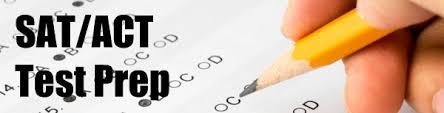Test prep students, when you are registering for live classes utilizing our Acuity online scheduler, please make sure that you are signing up for the correct class based on your strength test. You should be signing up for either ACT or SAT classes, not the other and not both. This confusion is causing classes to fill up in error. Also, please do not sign up more than once for each individual class as this is also causing classes to fill up in error. We truly appreciate your CAREFUL attention to this matter.

Please register for the ACT at act.org OR SAT at collegeboard.org. Please note there are early registration deadline dates. You can register after the deadline date but a late fee will be required.

 table div table+table+table+table+table+table+table+table+table+table+table+table+table+table+table+table+table+table+table+table+table+table+table+table+table+table+table+table+table+table+table+table+table+table+table+table+table+table+table+table+table+table+table+table+table div table{width:100%;padding:0}table div table+table+table+table+table+table+table+table+table+table+table+table+table+table+table+table+table+table+table+table+table+table+table+table+table+table+table+table+table+table+table+table+table+table+table+table+table+table+table+table+table+table+table+table+table div table img{width:96.23%;padding:0;float:none}table div table+table+table+table+table+table+table+table+table+table+table+table+table+table+table+table+table+table+table+table+table+table+table+table+table+table+table+table+table+table+table+table+table+table+table+table+table+table+table+table+table+table+table+table+table div table td{width:100%;padding:0 1.88% 18px}/* styles */## Scholarships Search Tools

 /* styles */ There are many good scholarship search tools/engines on the internet. Their primary function is to assist you in finding scholarships that match the eligibility criteria you enter for GPA, gender, residency, ethnicity/heritage, religion and area of study, hobbies, interests — some of the most common criteria used. The College Map team recommends the following search engines: Scholarships.com FastWeb Big Future Unigo SallieMae Please make sure to "click" or fill out as much information as possible. Once you've entered your information into the database, the search engine will generate a list of all current scholarships you qualify for. We recommend sorting that list by due date.
 table div table+table+table+table+table+table+table+table+table+table+table+table+table+table+table+table+table+table+table+table+table+table+table+table+table+table+table+table+table+table+table+table+table+table+table+table+table+table+table+table+table+table+table+table+table+table+table+table div table{width:100%;padding:0}table div table+table+table+table+table+table+table+table+table+table+table+table+table+table+table+table+table+table+table+table+table+table+table+table+table+table+table+table+table+table+table+table+table+table+table+table+table+table+table+table+table+table+table+table+table+table+table+table div table img{width:96.23%;padding:0;float:none}table div table+table+table+table+table+table+table+table+table+table+table+table+table+table+table+table+table+table+table+table+table+table+table+table+table+table+table+table+table+table+table+table+table+table+table+table+table+table+table+table+table+table+table+table+table+table+table+table div table td{width:100%;padding:0 1.88% 18px}/* styles */## Links and Resources

Our private "Your Path" page contains details for upcoming deadlines, events, and important announcements. We encourage you to check it regularly! The password is case-sensitive:
prepare@TCM2018

# Test Prep Schedules

Be sure to register for your test prep classes, available on the Your Path page and the Acuity Scheduler. Registration is mandatory; walk-ins may not be able to be accommodated.

# Suggestions?

Is there something you'd like to see in our newsletter? An event you'd like us to host? A friend you'd like to refer? Let us know by simply replying to this message.

 /* styles */ Kind regards, Your Team at The College Map